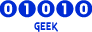## A distance issue between 2 monsters [MACRO]

Forum closed. All further discussion to be discussed at https://github.com/OpenKore/

Moderator: Moderators

Message
Author
ezza
DevelopersPosts: 109
Joined: 04 Apr 2008, 09:50

### A distance issue between 2 monsters [MACRO]

Last time I have upgrade my monster syntax in automacro to be like this --> Please Click Here. Then, suddenly I feels like the incremental value of \$counter (risk point) is not soo logic becoz, its just do the increament if the bot saw any same species of that particular monster on screen.
The increment happened even if the next monster to check (\$monster->name) is far away from our targeted monster. Which seems its not logic to me since the target monster risk shud not increase if the monster is far away from the target, rught?

So, now I've tried to do the increment if the distance from \$monsters{\$_} (target) to \$monster (in sight) is below 12 blocks. Here is the code...

monster Female Thief Bug,Thief Bug, 12

Code: Select all

``````sub checkMonster {
my (\$monsterList, \$mondist) = \$_ =~ /^(.*),?\s(\d+)\s*\$/;
return 0 if (!defined \$mondist || \$mondist < 1);
foreach (@monstersID) {
next unless defined \$_;
if (existsInList(\$monsterList, \$monsters{\$_}->{name})) {
my \$counter;
my \$mypos = calcPosition(\$char);
my \$pos = calcPosition(\$monsters{\$_});
my \$dist = sprintf("%.1f", distance(\$mypos, \$pos));
my \$val = sprintf("%d %d %s", \$pos->{x}, \$pos->{y}, \$field->{name});
\$varStack{".lastMonster"} = \$monsters{\$_}->{name};
\$varStack{".lastMonsterPos"} = \$val;
\$varStack{".lastMonsterDist"} = \$dist;
\$varStack{".lastMonsterID"} = \$monsters{\$_}->{binID};
for (my \$i = 0; \$i < @::monstersID; \$i++) {
next if \$::monstersID[\$i] eq "";
my \$monster = Actor::get(\$::monstersID[\$i]);
if (\$monster->name eq \$monsters{\$_}->{name}) {
if (\$monster->{binID} eq \$monsters{\$_}->{binID}) {
\$counter++;
next;
} else {
my \$monsToMonDist = sprintf("%.1f",distance(\$pos, \$monster->{pos_to}));
\$counter++ if \$monsToMonDist < 12;
next;
}
}
next;
}
\$varStack{".lastMonsterCount"} = \$counter;
return \$dist <= \$mondist ?1:0
}
}
return 0
}``````

Question:-
1. Is my distance between the 2 monsters (target and insight monster) correct by using below code?

Code: Select all

``````if (\$monster->name eq \$monsters{\$_}->{name}) {
if (\$monster->{binID} eq \$monsters{\$_}->{binID}) {
\$counter++;
next;
} else {
my \$monsToMonDist = sprintf("%.1f",distance(\$pos, \$monster->{pos_to}));
\$counter++ if \$monsToMonDist < 12;
next;
}
} ``````
If wrong or the code seems weird, pls enlighten me to correct my mistake.